# Electronics and Communication Engineering - Automatic Control Systems - Discussion

20.

A system with transfer function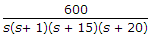may be approximated by the system

 [A].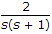[B].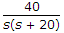[C].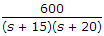[D].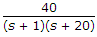Explanation:

Coefficient (s + 15) gives the term e-15t and the coefficient (s + 20) gives the term e-20t.

These can be neglected as compared to terms corresponding to s and (s + 1).

 R.Vignesh said: (Dec 27, 2018) Here, e Power minus infinity is zero so, the e power -15 t and e power -20 t is zero that's why it is neglected.